Krasnoshtanov A. P., Kupriyanova E. V., Protsykova M. A., Rakovskaia C.A., Slonova L.A.

Krasnoyarsk State University

Russia, Krasnoyarsk

THE IDENTIFICATIN OF MULTIPLY CONNECTED SYSTEMS UNDER INCOMPLETE INFORMATION

Annotation. The problems of interconnected stochastic systems identification in the broad sense are considered in the work. In addition the priory information about the parametric model structure is absent. Under these conditions the nonparametric modeling occurs to be perspective.

Красноштанов А. П., Куприянова E. В., Процыкова M. А., Раковская С. А., Слонова Л. А.

Сибирская Аэрокосмическая Академия

Красноярский Государственный Университет

Красноярский Государственный Торгово-экономический Институт

Россия, Красноярск

ИДЕНТИФИКАЦИЯ МНОГОСВЯЗНЫХ СИСТЕМ ПРИ НЕПОЛНОЙ ИНФОРМАЦИИ

Аннотация. В работе рассматриваются задачи идентификации взаимосвязанных стохастических систем в широком смысле. При этом априорная информация о параметрической структуре модели отсутствует. В этих условиях перспективным оказывается непараметрическое моделирование.

Let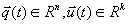be the vectors of observed random sequences representing accordingly output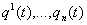and input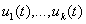variables of the analyzed process. Let's define the function of solutions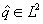as some function representing the output of the adaptive system in the influence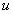.

For the open scheme, shown in the figure 1, following variables are introduced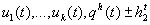which are observed in discrete moments of time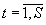of finite or infinite size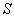.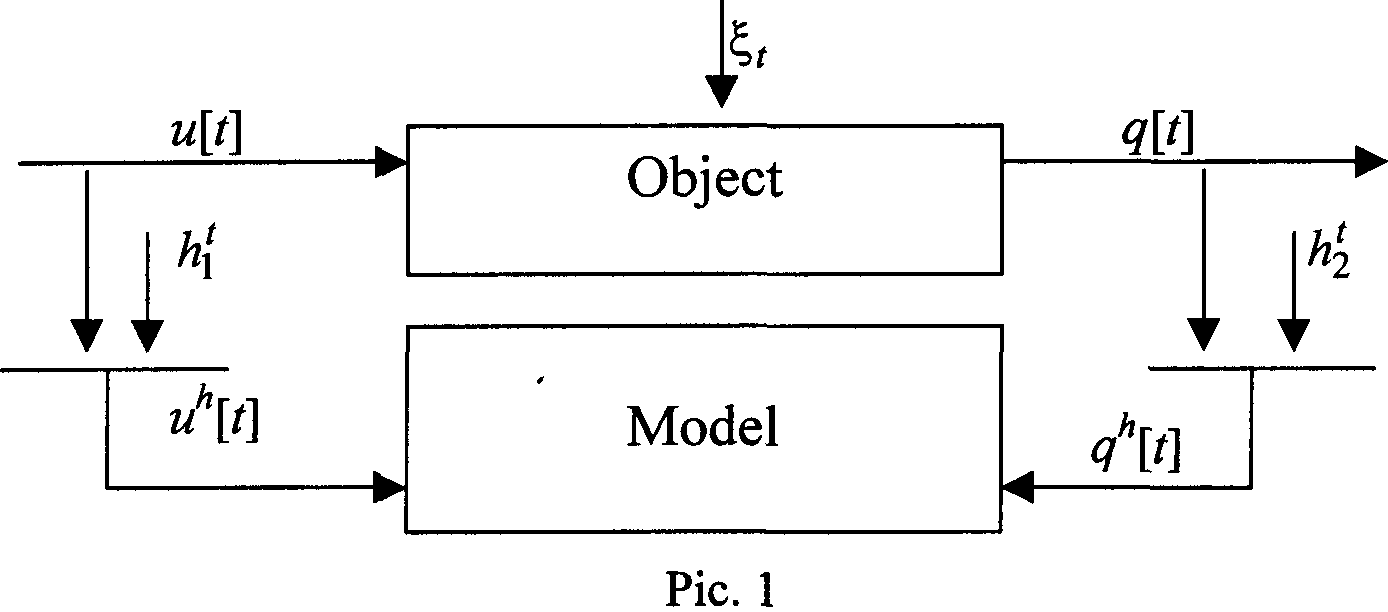Further index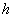in observed variables is omitted because of the simplicity reason. The noiseand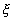, are such, that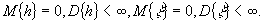Let's form the optimum criterion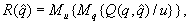where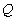is some convex function. The problem of searching of the best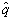amounts to the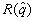minimization with respect tothat is the search if such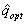that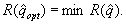It's clear that the form ofwill be determined be the form of function. If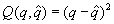then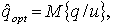(1)

We also may consider other types of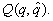Further the problem is in estimating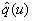by the observations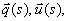which are formed out of the initial samples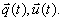The sign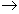denotes the time vector.

With the passive information accumulation, i.e. in the presence of the sample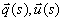the nonparametric estimating algorithm (1) is :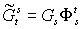(2)

where the matrices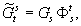are such, that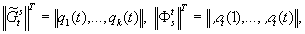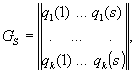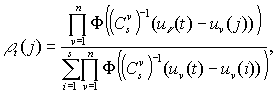Where T- transpose sign.

While constructing the model of some object the problem is in finding the estimate of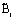function describing the object's behavior. This estimate is usually found by using the mean square optimum criterion.

It's clear that theestimate error mean square will be minimal if to takeas the mean of the random value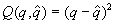in the giveni.e.represents the regression.

The construction of the regression models gives a good result in case of symmetric conditional probability densities, as since the mean value ofpractically coincides the most probable one. If the probability distribution has the brightly expressed asymmetric character then it is better to use the probability models. As the functionis the optimum criterion we'll take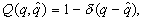where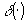, -the Dirac delta-function. After the minimization of this criterion with respect towe get the mode of conditional probability density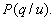, i.e.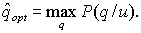(3)

As it is known the nonparametric estimate of conditional probability density is the estimate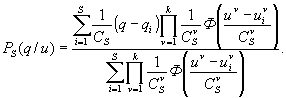(4)

Where the kernels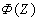are symmetric, delta-like for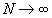functions with properties of probability density at the set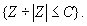It's important from the practical point of view to analyze the regression models in nonstationary (unstable) conditions. It's assumed that the probabilistic characteristics of an object are changing during the time in unknown way. The nonparametric estimate of regression function which looks like: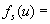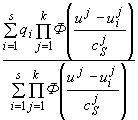(5)

and it should be modified by putting the additional weights - some kind of "memory functions", decreasing with the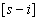increase. Ibis fact corresponds to "forgetting" the old information.

Essential interest, from the practical point of view, represents the case when for some relations between the vector components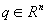and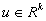the parametric relation is known up to the set of parameters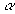, and other relations are unknown. In this situation we collide with the problem of identification which doesn't correspond neither to the parametric nor to the nonparametric level of priory information. These models arise when describing the complex interconnected processes (often this situation arises while modeling of manufacturing processes with the continuous technology) and has a form of an iterconnected system: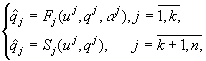, (6)

where the index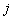at the arguments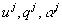denotes the definite (the-th) set of these components out of all components,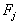-th parametric model,- nonparametric (the-th) model,-the vector of the output variables of the model,- the vector of the input variables,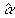-the parameters estimate.

The combined nonparametric models in the class of H-models look like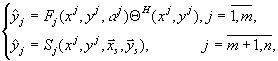(7)

where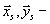the sample of statistically independent observations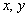of size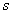at the domain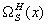of the set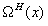. While using the H-models (7) the defects which are possible while using the models (6) are excluded. It's clear that if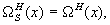then the models (7) transfer to the models (6). The setis called the domain of technological rule.

Let the manufacturing process be some aggregate of interconnected technological devices (objects), which models we'll denote as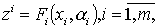where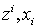-accordingly output and input variables of the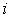-st object,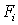-the parametric relation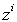on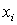, up to parameters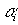and т - the number of objects. In case if all models are constructed (some models can be the models of nonparametric type), then the problem of model construction of the whole complex often arises. It's natural to use for mis purpose the models of local objects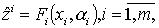of the type (6), (7). But arithmetic "assembling" of these models as the rule doesn't provide the desired accuracy. In techniques the analog of this fact is the assembling of some article of separate blocks each one of which satisfies the technical conditions but the functioning of the whole article doesn't satisfy the according technical specifications and that's why the necessity of the adjustment of the whole article arises, which represents the simultaneous adjustment of all or some local blocks. The process of "assembling" of the macro-model out of local ones and their following " adjustment" we called the models macro-synthesis.

LITERATURE

1. Medvedev A.V. Nonparametric adaptation systems. Novosibirsk: Nauka 1983

2. Tarasenko F.P. Nonparametric statistic. Tomsk: TGU, 1976.

 Site of Information Technologies Designed by  inftech@webservis.ru.